Quality and Process Methods > Measurement Systems Analysis > Statistical Details for Measurement Systems Analysis
Publication date: 07/30/2020

# Statistical Details for Measurement Systems Analysis

Intraclass Correlation without bias is computed as follows: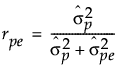Intraclass Correlation with bias is computed as follows: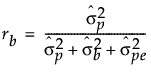Intraclass Correlation with bias and interaction factors is computed as follows: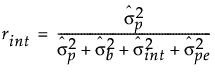Probable Error is computed as follows: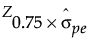Note the following: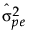= variance estimate for pure error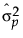= variance estimate for product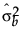= variance estimate for bias factors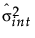= variance estimate for interaction factors

Z0.75 = the 75% quantile of standard normal distribution